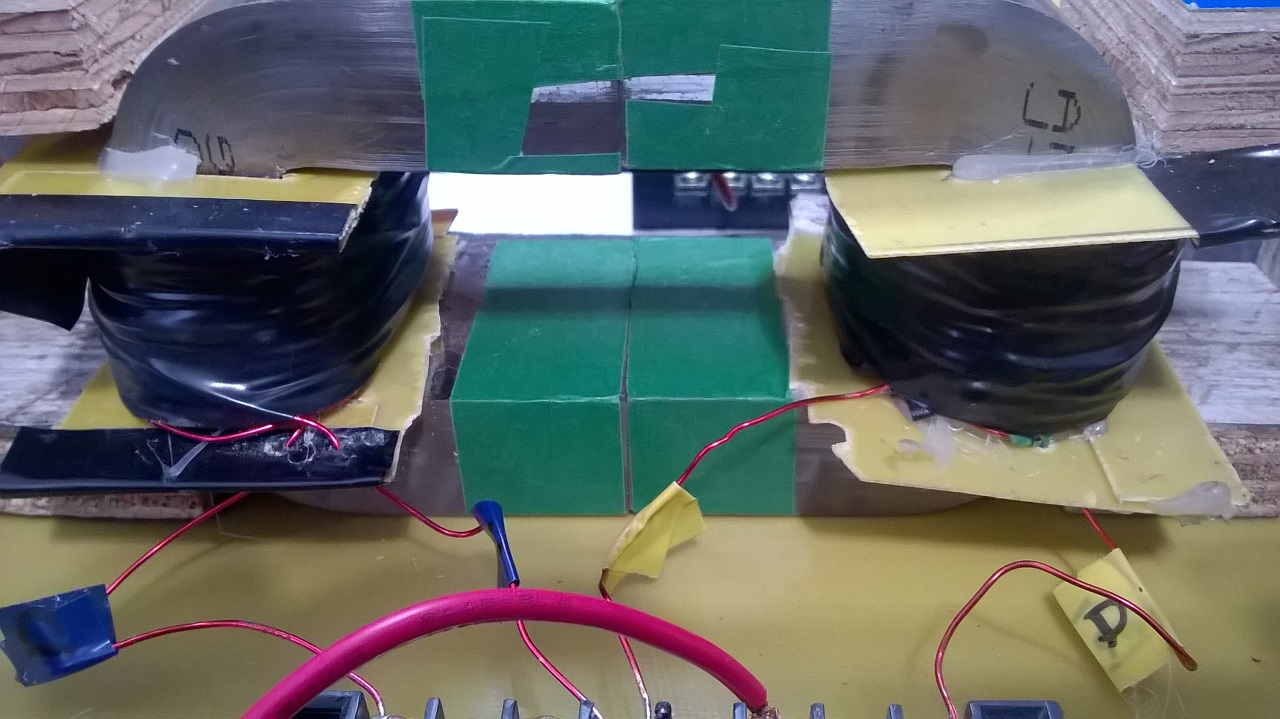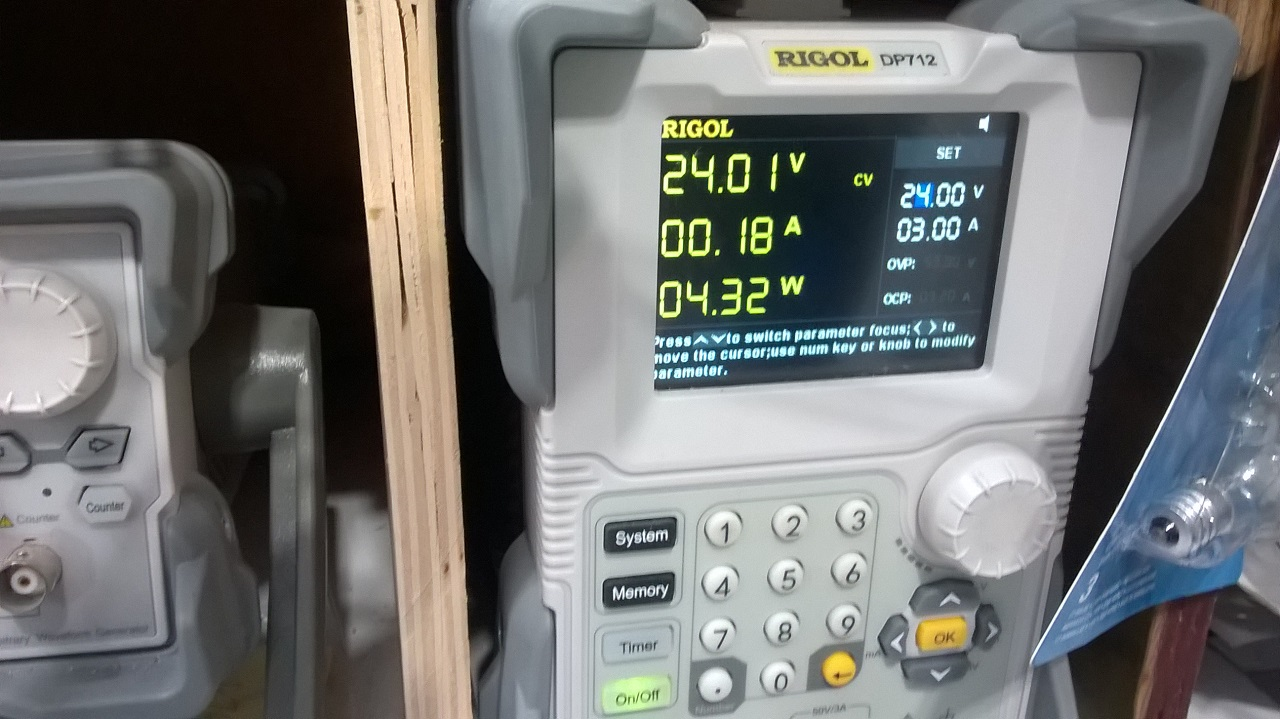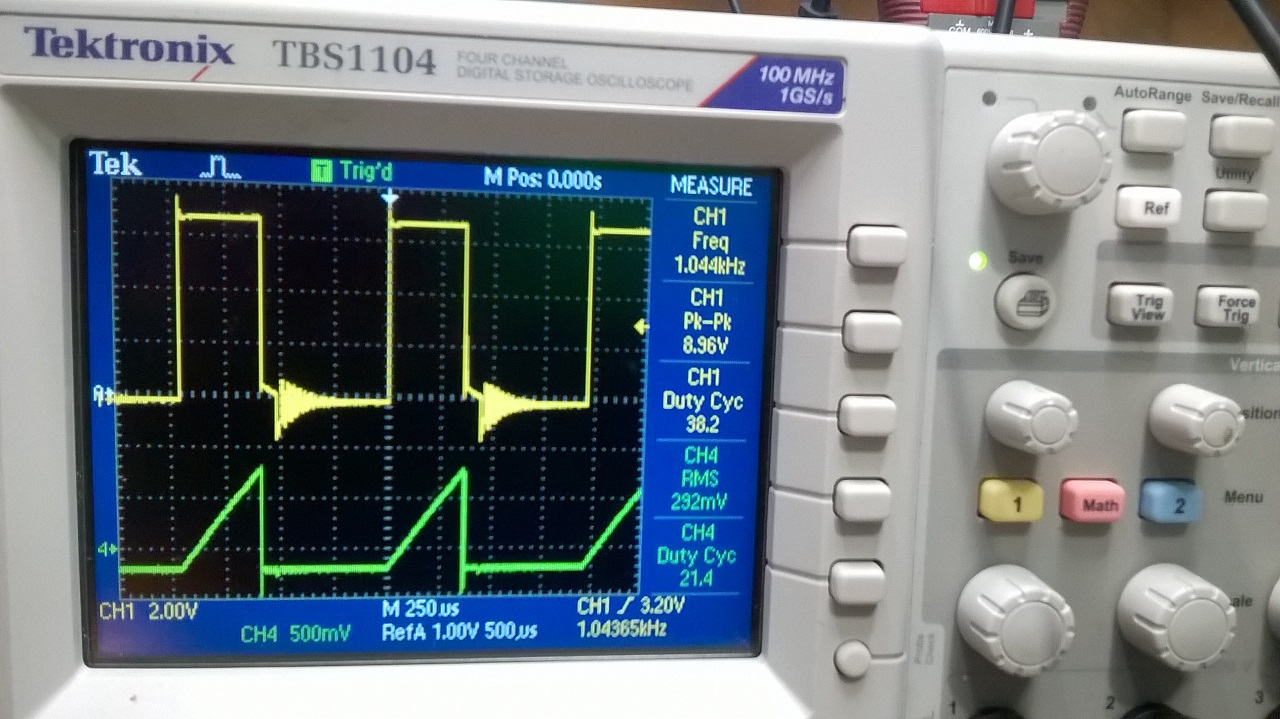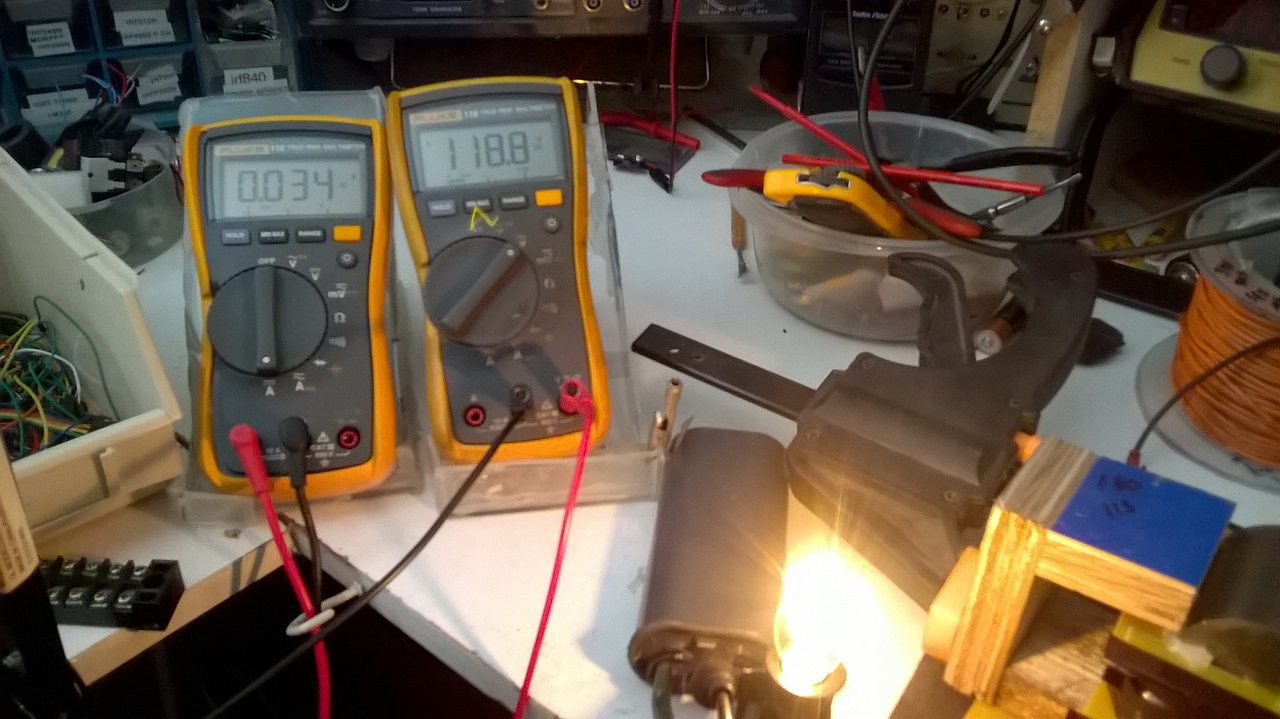My replication of Figther's ZPM. As my two coils were already mounted on an AMCC320 I was able to replicate quickly.
In the first image the 2 coils mounted on the AMCC320In the second image when the power supply is turned on at 24 volts DC he sees an input power of 4.32 watts on 24 volts and 180 ma when my TL494 oscillator is supplied to both coils through an IGBT pulsed at 38.2% on the high side. Channel1 is IGBT pulseImage of the oscilloscope with the current probe on the PSYou can notice that the oscilloscope show on a my TEK current probe (green) 292mv =292 ma  (rectangular form) and 38.2% DTC

Last image the 118 volt 4 Watts lamp lit with 34 ma x118.8v = therefore 4.0392 watts of power produce at the output on a large capacitor and 4 diodes in parallel in order to produce an almost pure DC which can be read by the 2 DDMsCalculation of input power in DCM mode with reactive component.

As indicated by the power supply we have an instantaneous pulse power of 4.32 watts, this is the instantaneous power not the average power over time.

V and I is what PS provided a DM as wrong lecture for input due to high frequency so to calc PIn we take well known formula for inductor.

Irms on scope is 0.292 Amp, DC voltage is 24volts

Pin= I*V*sqrt(Duty_Cycle)/sqrt(3)

Pin= 0.292 Amp x 24 Vdc x sqrt 0.382 / sqrt 3 = 2.533 watts

So for  Pout of 4.032 watts  i need only 2.533 watts at input, Still a pretty good COP
Jagau